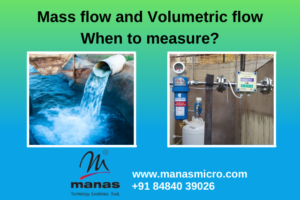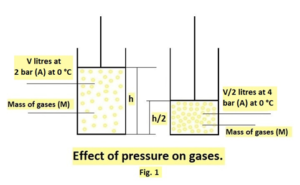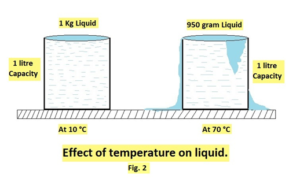# Mass flow and Volumetric flow

Mass flow and Volumetric flow – When to measure?

In the last article, we discussed how it is important to measure fluid flow. Here we will have a look at the difference between mass flow and volumetric flow. We will also discuss when to check the mass flow and when volumetric flow measurement is sufficient.What is Mass Flow Rate?
It is the total molecular mass passing through a cross-section of a given pipeline per unit of time.
Qm = Vm.Ap / T
Where,
Qm is the mass flow rate in Kg/sec.
Vm is average, point mass velocity in kg/sec.sq.m
Ap is the cross-sectional area of a given pipe in sq. meter.
T is the time in seconds.

What is Volumetric Flow Rate?
It is the total volume occupied by the molecular mass that passes through the cross-section of a given pipeline per unit time.
Qv = V.Ap / T
Where,
V is the average, point velocity.

How to measure Gas or Air Flowrate?
The gaseous fluids are compressible fluids, so their volume varies with pressure and
temperature. When the pressure increases, the volume decreases and when the pressure decreases, the volume increases. When temperature increases, the volume also increases and vice versa.

In this case, the volumetric flow rate or the so-called actual flow rate does
not deliver any useful information unless the pressure and temperature are specified.
It is a standard practice to express mass flow in terms of volumetric flow rate under Standardized or Normalized conditions of pressure and temperature. It means the volume occupied by the given mass of gas under STP or NTP conditions.To avoid the problem in measurement, as shown above, there are some standard methods for defining the right volume of gaseous fluids.
The volume,
Vol a P(A) / T(A), where, P(A) is the absolute pressure and T(A) is the absolute temperature.
P(A) = Pg + Atmospheric pressure.
T(A) = (temp.)°C + 273.15
Pg is gauge pressure measured with respect to atmospheric pressure, in Bar or Kpa.
There are two base conditions, a) NTP condition 2) STP condition.
NTP means normalized pressure and temperature.
It means 0°C and 1-atmosphere pressure (101.325 Kpa).
STP means 15.5°C and 1 Atmosphere pressure
many people define standard temperature as 21.1°C 0

Here are some fun facts about STP and NTP conditions

For Compressed Air, Compressed Nitrogen, etc. the standard temperature is taken as 21.10 C
The volumes are then specified in Sm3(Standardized cubic meters)
or Nm3 (Normalized cubic meters).

In some countries, the definitions of STP and NTP conditions are reversed.

E.g. in US STP means 00 C and NTP means 21.10 or 200 C while as in Europe it is reverse.

These NTP or STP conditions are called Base Conditions. It is better to get clarified with the user/manufacturer the way they define Base Conditions.

Manas Air/Gas Flow Meters (Scirocco 1000 series) have a facility to switch to any desired base condition, as mentioned above.

How to measure Liquid or Water Flow rate?

Liquids are not compressible fluids and variation of volume with usual pressure values is not measurable. The volume of the liquid varies with temperature and has to be considered whenever precise calculations of flow rate are essential.

However, the thermal expansion coefficient for volume is much less for liquids as compared to gases hence usually no remarkable difference occurs in volume flow rate with a variety of fluid temperature. Therefore, with few exceptions, its effect is not considered.Many instrument experts who have the knowledge of process also, claim that in a majority of cases the selection of flow meters in the field is not correct. What the process needs is different from what the instrument delivers.

The coordination between a process expert and an instrument expert is very important in the selection of the proper flow meter in the proper place. Sometimes an experienced person is not aware of the new technology as he never has come across such technology in the past.

Basic types of Mass Flow Meters

There are two types of mass flow meters.

• Direct measurement of Mass flow rate.
• Indirect method of measuring mass flow rate.

Meters based on Coriolis principle or Thermal Dispersion principle are Direct Mass flow meters.

Meters based on turbine, electromagnetic, differential pressure, etc. principle can be converted to Indirect Mass Flow Meters.

The direct mass flow measuring instruments are very expensive. However, there are some reliable methods to find mass flow indirectly. This is quite inexpensive. Wherever feasible, people prefer these methods. Indirect mass flow using a pressure transmitter and a temperature transmitter to determine the density of flowing fluid. Mass flow measurement is then achieved by determining the variations in density with temperature and pressure.

Qm = Qv.r, Where r is the flowing density of the fluid.

This method is called Indirect Mass flow measurement. This method needs additional measurement of fluid pressure and fluid temperature. This whole system becomes less accurate compared to Direct Mass Flow Measurement. Yet it is reliable and is acceptable for many applications where very high accuracy levels are not required.

Manas offers, both Thermal Dispersion(Direct) Mass Flow Meters and Orifice based(Indirect) Mass Flow Meters, depending on the customer’s need and application.